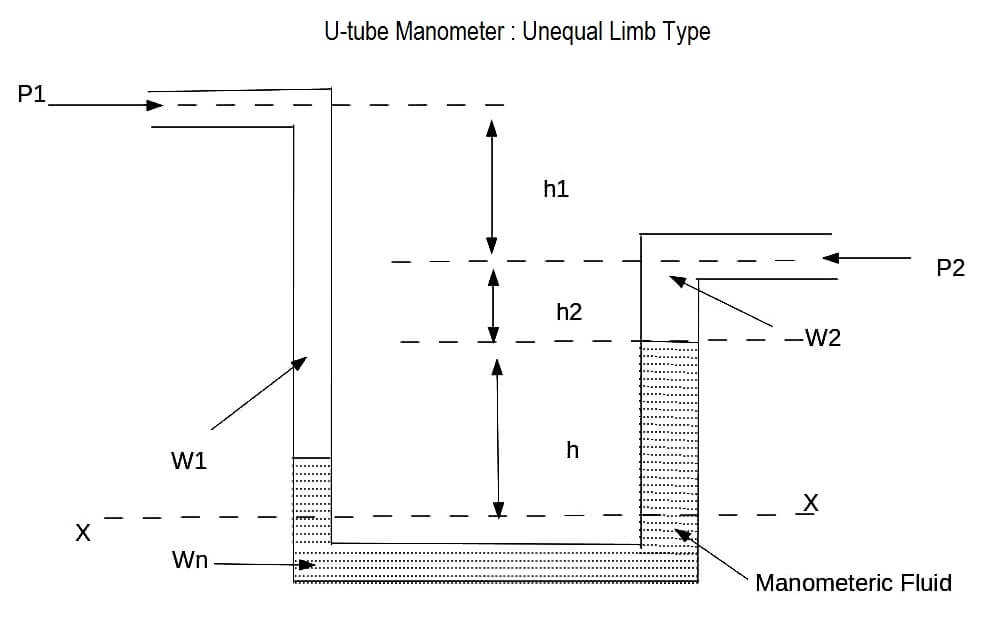# U-tube Manometer with equal & unequal limb types

This is the most simple and precise device used for the measurement of pressure.

It consists of a transparent tube constructed in the form of an elongated ‘U’, and partially filled with the manometeric fluid such as mercury. The purpose of using mercury as the manometeric fluid is that their specific gravity at various temperatures are known exactly and they dont stick to the tube. The two common types of manometers are the equal limb type and unequal limb type.

#### Operation of Manometer :

To measure the pressure of a fluid which is less dense and immersible with the manometeric fluid, it is applied to the top of one of the limbs of the manometer while a reference fluid pressure (generally atmospheric ) is applied to the other limb.

Let : P1 = unknown pressure with specific weight w1.
And : P2 = reference pressure with specific weight w2.
And : wm = specfic weight of the manometeric fluid.

The difference between the pressure on the two limbs of the manometer is a function of ‘h’, the difference between the levels of the manometeric fluid in the two limbs. ‘h’, can be read directly by placing a scale near the manometer.

#### For Equal Limb type:The pressure balance equation is

P1 + gh * w1 = P2 + gh * wm

therefore, differential pressure

P = P1 – p2 = gh(wm – w1)

#### For unequal Limb typeThe pressure balance equation is : (section-XX)

P1+w1(h1+h2+h) = P2 + w2h2 + wmh

Therefore, differential pressure:

P = P1 – P2 = w2h2 + wm * h – w1(h1 + h2 + h)

= wm*h [ 1 + (w2/wm * h2/h) – w1/wm ( h1+h2/h + 1) ]

= wmh * Cn

where; Cn = [ 1 + ( w2/wm – h2/h) – w1/wm ( h1+h2/h + 1)]

Cn is known as hydraulic correction factor.

#### Applications of Manometer

• they are used to sense differential pressure in venturi meter and other flow meters.
• They are used as level devices to sense liquid heads.
• There are used as primary standard for pressure measurements.

• They are simple is construction.
• They give accurate results.
• Wide range of manometeric fluids ar available such as mercury, water, aniline, tetrabromethane, bromoform and carbon tetrachloride.